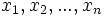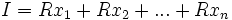# Finitely generated ideal

This article defines a property of an ideal in a commutative unital ring |View other properties of ideals in commutative unital rings
This property of ideals in commutative unital rings depends only on the ideal, viewed abstractly as a module over the commutative unital ring. The corresponding module property that the ideal must satisfy is: finitely generated module
View other such properties

## Definition

### Symbol-free definition

An ideal in a commutative unital ring is said to be finitely generated if it has a finite generating set, that is, if there is a finite set such that it is the smallest ideal containing that finite set.

### Definition with symbols

An ideal$I$ in a commutative unital ring$R$ is said to be finitely generated if there is a finite set$x_1, x_2, ..., x_n$ such that$I = Rx_1 + Rx_2 + ... + Rx_n$.

## Metaproperties

### Trimness

This property of ideals in commutative unital rings is trim: it is satisfied by the zero ideal and by the whole ring, as an ideal of itselfTemplate:Finite-sum-closed ideal property

### Intersection-closedness

This property of ideals in commutative unital rings is not closed under taking arbitrary intersections; in other words, an arbitrary intersection of ideals with this property need not have this property

In general, an intersection of finitely generated ideals need not be finitely generated. However, for Noetherian rings, where every ideal is finitely generated, an intersection of finitely generated ideals is certainly finitely generated.